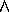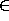Next: General Operations Up: A Weakest Precondition Calculus Previous: Analysis of Authentication Protocols

# Weakest Preconditions

This section describes the wps for each of the authentication operations. In general, a wp acts as a textual substitution which replaces one condition with another set of equivalent conditions. The wps for each operation are presented in tables of three columns: the postcondition, the weakest precondition necessary for the postcondition, and the reasoning. Axiom numbers refer to SvO axioms in [SvO94].

The wps depend in part upon which postconditions are desired. Some postconditions are true, regardless of the preconditions, as long as the operation is performed. For example, the operation ``B receives X'' makes the postconditions ``B receives X'' and ``B sees X'' automatically true. Similarly, some preconditions are required regardless of what postconditions are desired. For example, the operation ``B sends X'' has as a required precondition ``A sees X'' which must be true. These required new weakest preconditions are marked with a star.

Many of the weakest preconditions have corresponding conditions regarding the beliefs of the active party. The corresponding conditions for belief are derived using the same axiom as the original substitution plus necessitation. For example, the weakest preconditions of ``fresh(X)'' are ``fresh(X)XX'' by Axiom 17 and thus for beliefs the weakest preconditions of ``B believes (fresh(X))'' are ``B believes (fresh(X))XX'', by Axiom 17 and necessitation. Note that necessitation may only be used in conjunction with theorems and not derivations [SvO96].

Jim Alves-Foss
Fri Aug 1 16:00:31 PDT 1997